# 2 2 Notes Derived Units Density Derived units

• Slides: 122. 2 – Notes – Derived Units Density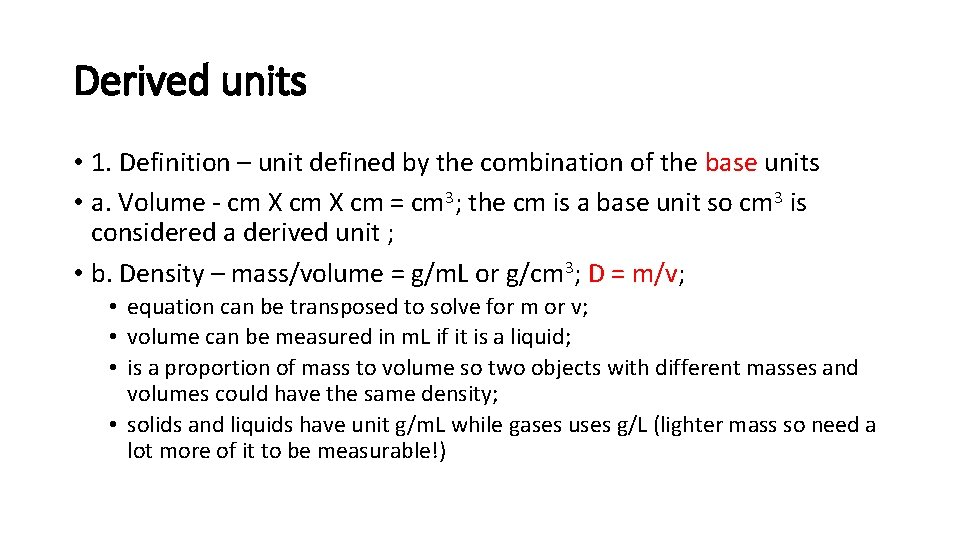Derived units • 1. Definition – unit defined by the combination of the base units • a. Volume - cm X cm = cm 3; the cm is a base unit so cm 3 is considered a derived unit ; • b. Density – mass/volume = g/m. L or g/cm 3; D = m/v; • equation can be transposed to solve for m or v; • volume can be measured in m. L if it is a liquid; • is a proportion of mass to volume so two objects with different masses and volumes could have the same density; • solids and liquids have unit g/m. L while gases uses g/L (lighter mass so need a lot more of it to be measurable!)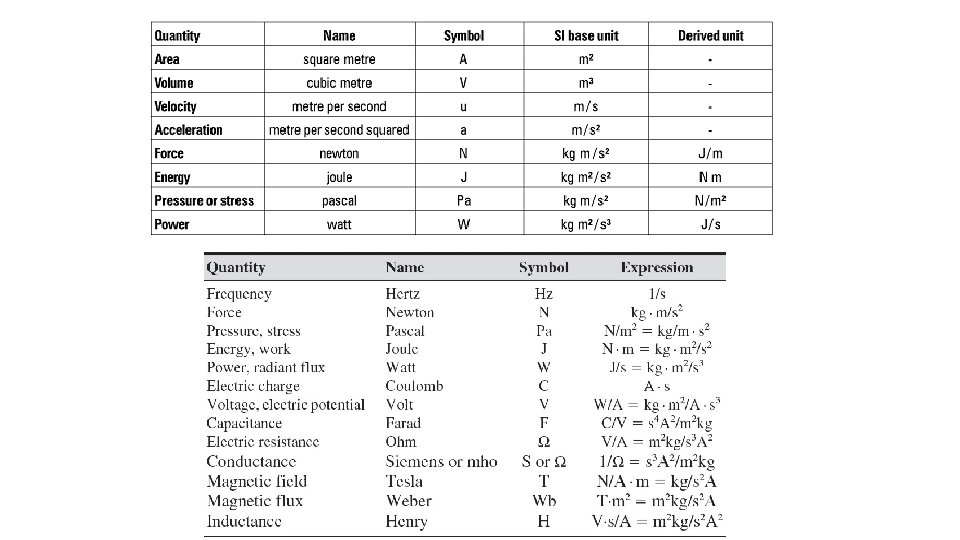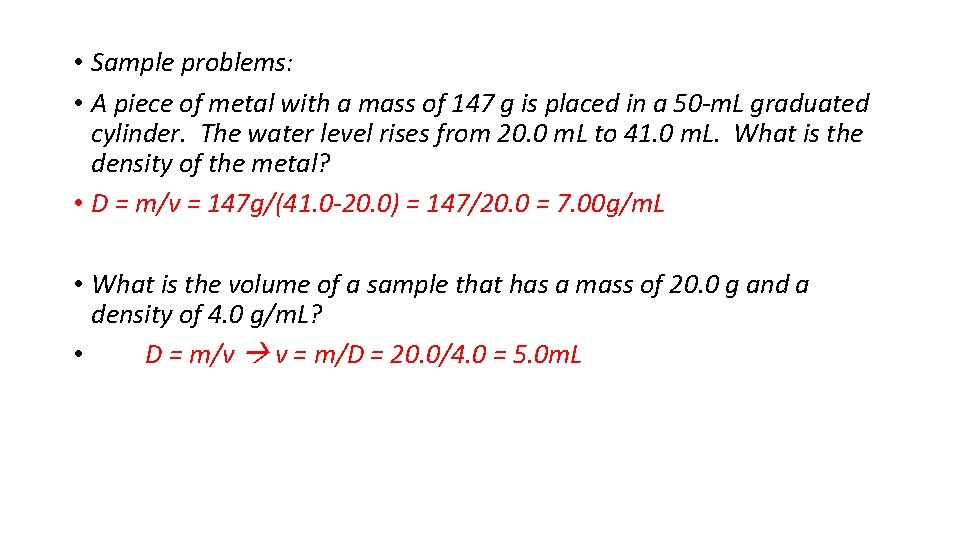• Sample problems: • A piece of metal with a mass of 147 g is placed in a 50 -m. L graduated cylinder. The water level rises from 20. 0 m. L to 41. 0 m. L. What is the density of the metal? • D = m/v = 147 g/(41. 0 -20. 0) = 147/20. 0 = 7. 00 g/m. L • What is the volume of a sample that has a mass of 20. 0 g and a density of 4. 0 g/m. L? • D = m/v v = m/D = 20. 0/4. 0 = 5. 0 m. L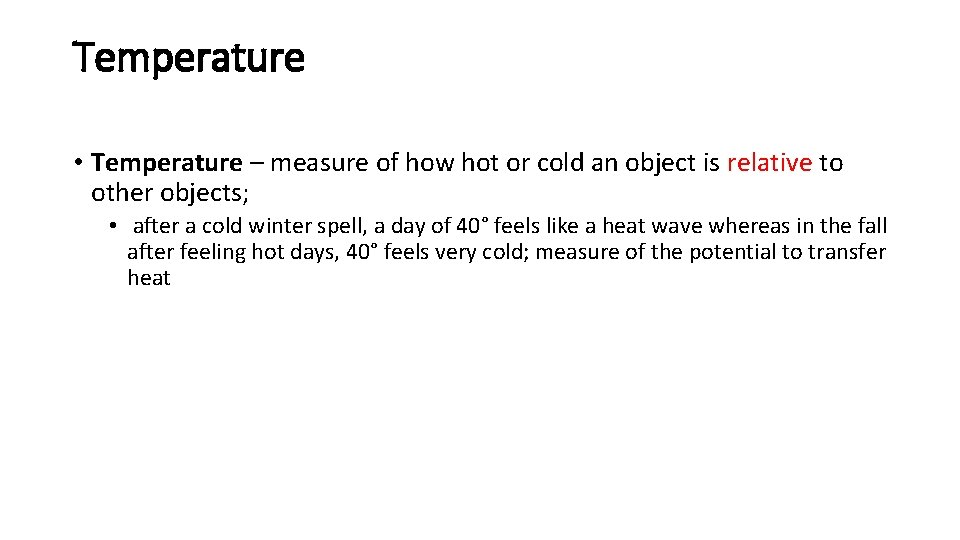Temperature • Temperature – measure of how hot or cold an object is relative to other objects; • after a cold winter spell, a day of 40° feels like a heat wave whereas in the fall after feeling hot days, 40° feels very cold; measure of the potential to transfer heat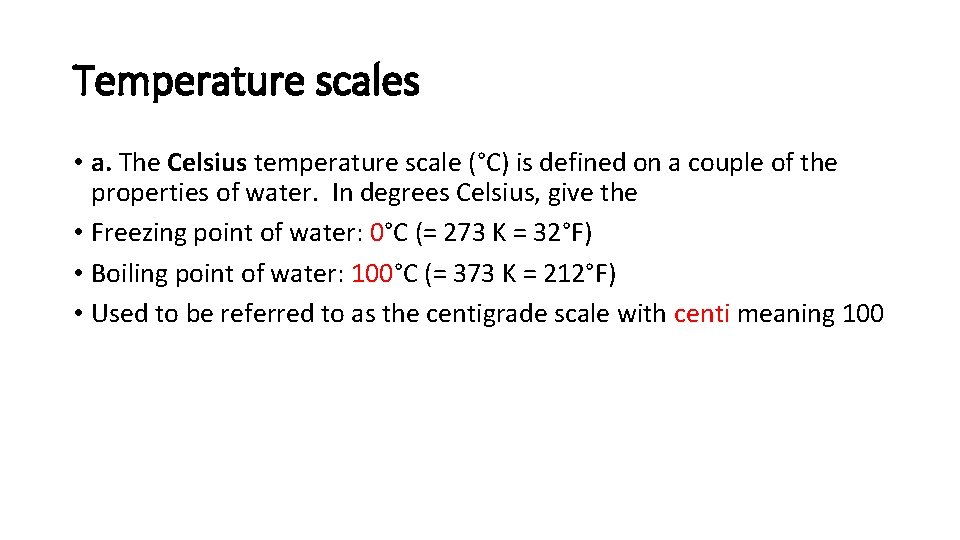Temperature scales • a. The Celsius temperature scale (°C) is defined on a couple of the properties of water. In degrees Celsius, give the • Freezing point of water: 0°C (= 273 K = 32°F) • Boiling point of water: 100°C (= 373 K = 212°F) • Used to be referred to as the centigrade scale with centi meaning 100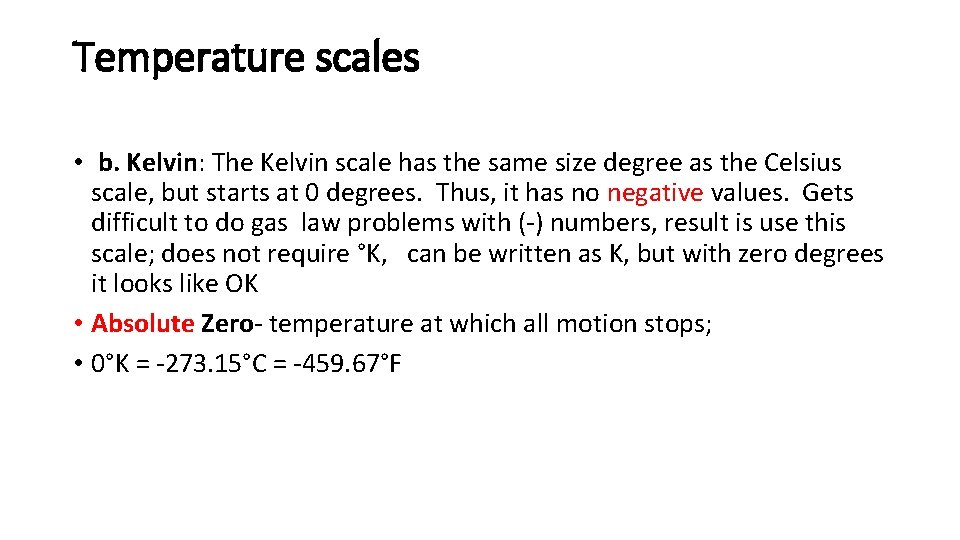Temperature scales • b. Kelvin: The Kelvin scale has the same size degree as the Celsius scale, but starts at 0 degrees. Thus, it has no negative values. Gets difficult to do gas law problems with (-) numbers, result is use this scale; does not require °K, can be written as K, but with zero degrees it looks like OK • Absolute Zero- temperature at which all motion stops; • 0°K = -273. 15°C = -459. 67°FTemperature scales • c. Fahrenheit: English temperature scale; not used in chemistry; parallels a 4 th temperature scale – Rankin; R = °F + 459. 67; will make all Fahrenheit temperatures (+) • *Rankin scale- has absolute zero as 0, but 1 degree is equal to 1 degree F.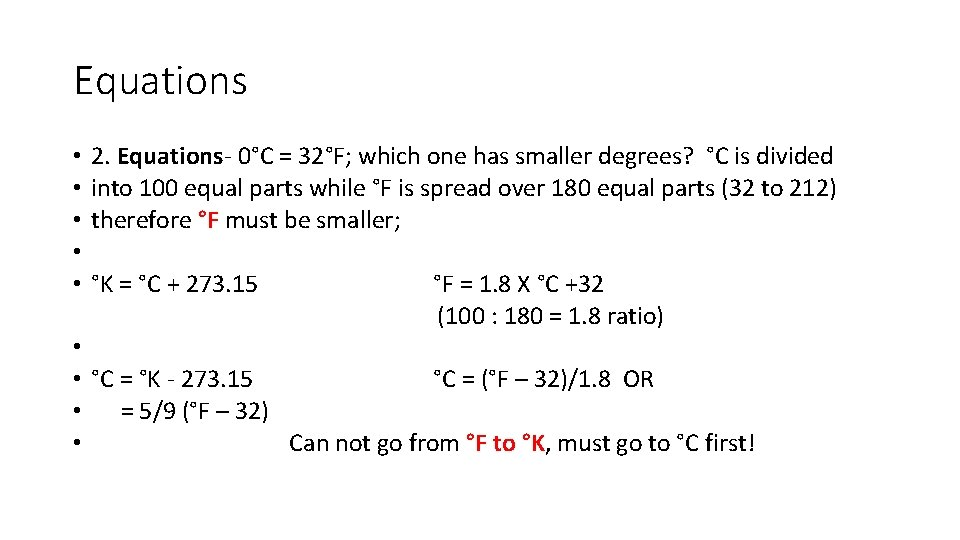Equations • 2. Equations- 0°C = 32°F; which one has smaller degrees? °C is divided • into 100 equal parts while °F is spread over 180 equal parts (32 to 212) • therefore °F must be smaller; • • °K = °C + 273. 15 °F = 1. 8 X °C +32 (100 : 180 = 1. 8 ratio) • • °C = °K - 273. 15 °C = (°F – 32)/1. 8 OR • = 5/9 (°F – 32) • Can not go from °F to °K, must go to °C first!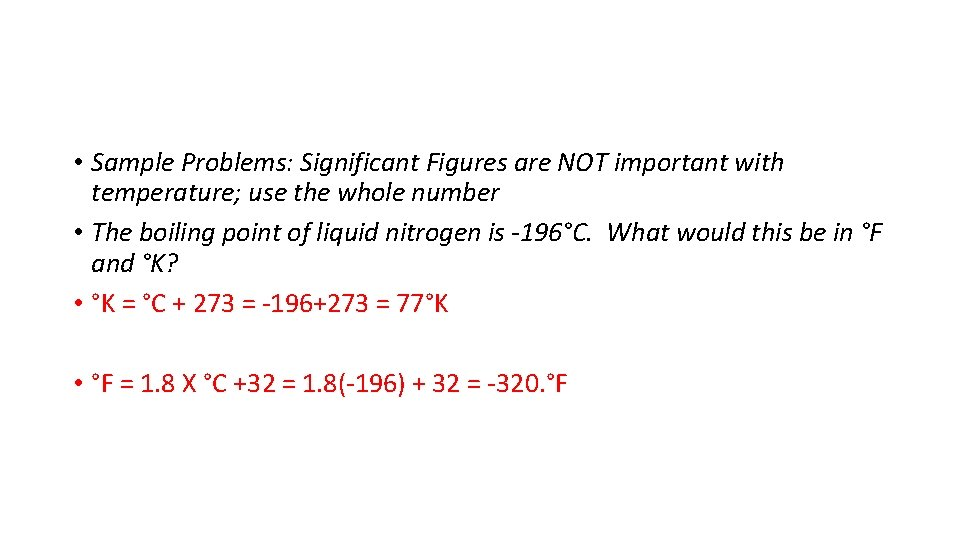• Sample Problems: Significant Figures are NOT important with temperature; use the whole number • The boiling point of liquid nitrogen is -196°C. What would this be in °F and °K? • °K = °C + 273 = -196+273 = 77°K • °F = 1. 8 X °C +32 = 1. 8(-196) + 32 = -320. °F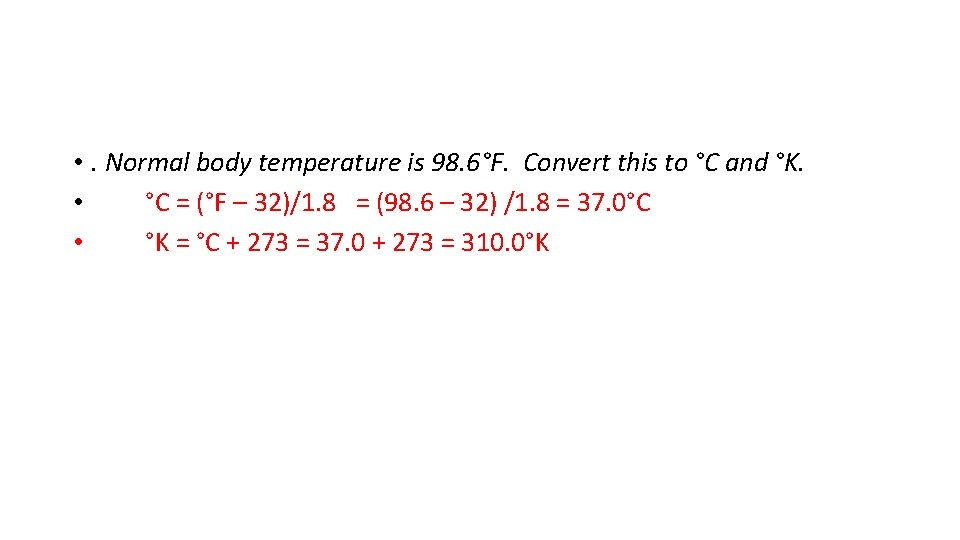• . Normal body temperature is 98. 6°F. Convert this to °C and °K. • °C = (°F – 32)/1. 8 = (98. 6 – 32) /1. 8 = 37. 0°C • °K = °C + 273 = 37. 0 + 273 = 310. 0°K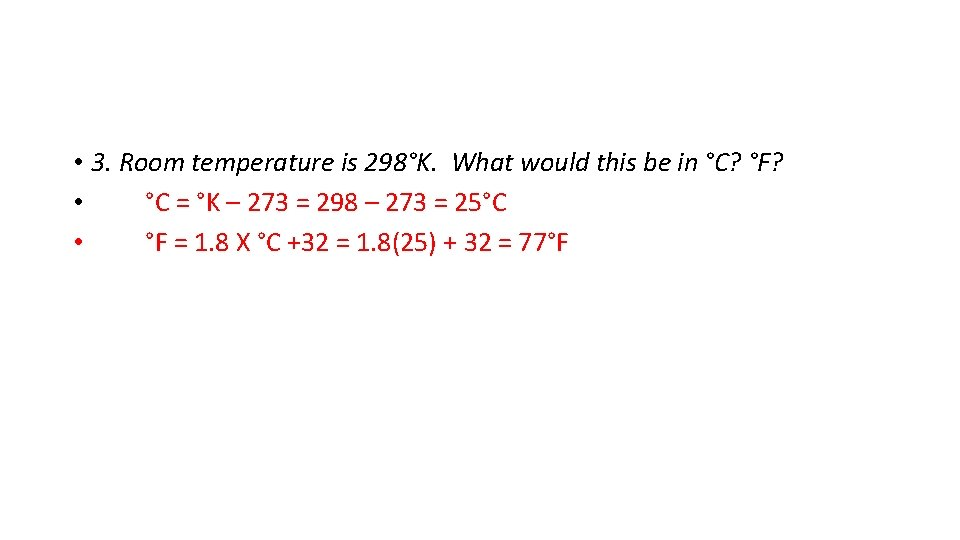• 3. Room temperature is 298°K. What would this be in °C? °F? • °C = °K – 273 = 298 – 273 = 25°C • °F = 1. 8 X °C +32 = 1. 8(25) + 32 = 77°F